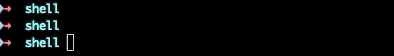2022
04-27

# 如何用c语言编写秒表(c语言怎么计时输出秒)#### 实现思路及代码 如何获取流逝的时间长度？

```
begin_at=\$(date '+%s') # 睡个觉 end_at=\$(date '+%s')
((interval=\${end_at} - \${begin_at}))
```

#### 如何换算为时分秒？

```
((hours=\${interval} / 3600)) ((minutes=(\${interval} % 3600) / 60)) ((seconds=(\${interval} % 3600) % 60))
```

#### 如何输出形如hh:mm:ss的格式？

hh:mm:ss的意思是分别用两个十进制数字显示时分秒，并以冒号分隔它们。如果有任何一个单位的数值小于 10，便用字符0填充左侧的空白。按这个格式，凌晨 1 点 2 分 3 秒便会显示为01:02:03。

```
hours=1
minutes=2
seconds=3

if [ "\${hours}" -lt '10' ];
then
echo -n "0\${hours}"
else
echo -n "\${hours}"
fi
echo -n ':'
if [ "\${minutes}" -lt '10' ];
then
echo -n "0\${minutes}"
else
echo -n "\${minutes}"
fi
echo -n ':'
if [ "\${seconds}" -lt '10' ];
then
echo -n "0\${seconds}"
else
echo -n "\${seconds}"
fi
```

```
printf "%02d:%02d:%02d" \${hours} \${minutes} \${seconds}
```

```
如何覆盖已经打印的内容？

只要用tput命令即可，示例代码如下

echo -n '11:22:33'
tput cr
tput el
echo '44:55:66'

关于cr和el，以及更多可以传给tput命令的参数，可以参见terminfo的man文档。

如何每隔一秒钟输出一次？

这大概是整个程序中最简单的需求了

while [ 1 -eq 1 ]
do
# 此处可以为所欲为
sleep 0.5
done

完整的秒表实现

至此，完整的秒表程序就可以实现出来了

#!/bin/bash
# 秒表，以hh:mm:ss的格式展示数据

begin_at=\$(date '+%s')

while [ 1 -eq 1 ]
do
end_at=\$(date '+%s')
# 算术运算：http://faculty.salina.k-state.edu/tim/unix_sg/bash/math.html
((interval=\${end_at} - \${begin_at}))
((hours=\${interval} / 3600))
((minutes=(\${interval} % 3600) / 60))
((seconds=(\${interval} % 3600) % 60))
tput cr
tput el
printf "%02d:%02d:%02d" \${hours} \${minutes} \${seconds}
sleep 0.5
done

运行后的效果如下：以上就是“如何用c语言编写秒表(c语言怎么计时输出秒)”的详细内容，想要了解更多C语言教程欢迎持续关注编程学习网

```